This Rust implementation embodies the latest implementation Adapton, which offers a foundational, language-based semantics for general-purpose incremental computation.

Programming model

• The documentation below gives many illustrative examples, with pointers into the other Rust documentation.
• The engine module gives the core programming interface.

Background

Adapton proposes the demanded computation graph (or DCG), and a demand-driven change propagation algorithm. Further, it proposes first-class names for identifying cached data structures and computations.

The following academic papers detail these technical proposals:

Why Rust?

Adapton's first implementations used Python and OCaml; The latest implementation in Rust offers the best performance thus far, since (1) Rust is fast, and (2) traversal-based garbage collection presents performance challenges for incremental computation. By liberating Adapton from traversal-based collection, our empirical results are both predictable and scalable.

• The Editor role creates and mutates input, and demands the output of incremental computations in the Archivist role.

• The Archivist role consists of Adapton thunks, where each is cached computation that consumes incremental input and produces incremental output.

Examples: The examples below illustrate these roles, in increasing complexity:

Programming primitives: The following list of primitives covers the core features of the Adapton engine. Each primitive below is meaningful in each of the two, editor and archivist, roles:

• Ref cell allocation: Mutable input (editor role), and cached data structures that change across runs (archivist role).
• Observation and demand: Both editor and archivist role.
• Thunk Allocation: Both editor and archivist role.
• Thunk allocation, without demand:
• Thunk allocation, with demand:

Start the DCG engine

The call init_dcg() below initializes a DCG-based engine, replacing the Naive default engine.

fn main() {
manage::init_dcg();

// Put example code below here
}

Create incremental cells

Commonly, the input and intermediate data of Adapton computations consists of named reference cells. A reference cell is one variety of Arts; another are thunks.

Implicit counter for naming cells

cell!(123) uses a global counter to choose a unique name to hold 123. Important note: This may be appopriate for the Editor role, but is never appropriate for the Archivist role.

let c : Art<usize> = cell!( 123 );

assert_eq!( get!(c), 123 );

Explicitly-named cells

Sometimes we name a cell using a Rust identifier. We specify this case using the notation [ name ], which specifies that the cell's name is a string, constructed from the Rust identifer name:

let c : Art<usize> = cell!([c] 123);

assert_eq!(get!(c), 123);

Optionally-named cells

Most generally, we supply an expression optional_name of type Option<Name> to specify the name for the Art. This Art is created by either cell or put, in the case that optional_name is Some(name) or None, respectively:

let n : Name = name_of_str(stringify!(c));
let c : Art<usize> = cell!([Some(n)]? 123);

assert_eq!(get!(c), 123);

let c = cell!([None]? 123);

assert_eq!(get!(c), 123);

Observe Arts

The macro get! is sugar for engine::force!, with reference introduction operation &:

let c : Art<usize> = cell!(123);

assert_eq!( get!(c), force(&c) );

Since the type Art<T> classifies both cells and thunks, the operations force and get! can be used interchangeably on Art<T>s that arise as cells or thunks.

Mutate input cells

One may mutate cells explicitly, or implicitly, which is common in Nominal Adapton.

The editor (implicitly or explicitly) mutates cells that hold input and they re-demand the output of the archivist's computations. During change propagation, the archivist mutates cells with implicit mutation.

Implicit mutation uses nominal allocation: By allocating a cell with the same name, one may overwrite cells with new content:

let n : Name = name_of_str(stringify!(c));
let c : Art<usize> = cell!([Some(n.clone())]? 123);

assert_eq!(get!(c), 123);

// Implicit mutation (re-use cell by name `n`):
let d : Art<usize> = cell!([Some(n)]? 321);

assert_eq!(d, c);
assert_eq!(get!(c), 321);
assert_eq!(get!(d), 321);

No names implies no effects: Using None to allocate cells always **gives distinct cells, with no overwriting:

let c = cell!([None]? 123);
let d = cell!([None]? 321);

assert_eq!(get!(c), 123);
assert_eq!(get!(d), 321);

Explicit mutation, via set: If one wants mutation to be totally explicit, one may use set:

let n : Name = name_of_str(stringify!(c));
let c : Art<usize> = cell!([Some(n)]? 123);

assert_eq!(get!(c), 123);

// Explicit mutation (overwrites cell `c`):
set(&c, 321);

assert_eq!(get!(c), 321);

Demand-driven change propagation

The example below demonstrates demand-driven change propagation, which is unique to Adapton's DCG, and its approach to incremental computation. The example DCG below consists of two kinds of nodes:

• Cells consist of data that changes over time, including (but not limited to) incremental input.

• Thunks consist of computations whose observations and results are cached in the DCG.

The simple example below uses two mutable input cells, num and den, whose values are used by an intermediate subcomputation div that divides the numerator in num by the denominator in den, and a thunk check that first checks whether the denominator is zero (returning zero if so) and if non-zero, returns the value of the division:

// Two mutable inputs, for numerator and denominator of division
let num = cell!(42);
let den = cell!(2);

// In Rust, cloning is explicit:
let den2 = den.clone(); // clone _global reference_ to cell.
let den3 = den.clone(); // clone _global reference_ to cell, again.

// Two subcomputations: The division, and a check thunk with a conditional expression
let div   = thunk![ get!(num) / get!(den) ];
let check = thunk![ if get!(den2) == 0 { None } else { Some(get!(div)) } ];

After allocating num, den and check, the editor changes den and observes check, inducing the following change propagation behavior. In sum, whether div runs is based on demand from the Editor (of the output of check), and the value of input cell den, via the condition in check:

1. When the editor demands thunk check the first time, Adapton executes the condition, and cell den holds 2, which is non-zero. Hence, the else branch executes get!(div), which demands the output of the division, 21.

2. After this first observation of check, the editor changes cell den to 0, and re-demands the output of thunk check. In response, Adapton's change propagation algorithm first re-executes the condition (not the division), and the condition branches to the then branch, resulting in None; in particular, it does not re-demand the div node, though this node still exists in the DCG.

3. Next, the programmer changes den back to its original value, 2, and re-demands the output of check. In response, change propagation re-executes the condition, which re-demands the output of div. Change propagation attempts to "clean" the div node before re-executing it. To do so, it compares its last observations of num and den to their current values, of 42 and 2, respectively. In so doing, it finds that these earlier observations match the current values. Consequently, it reuses the output of the division (21) without having to re-execute the division.

// Observe output of `check` while we change the input `den`
// Editor Step 1: (Explained in detail, below)
assert_eq!(get!(check), Some(21));

// Editor Step 2: (Explained in detail, below)
set(&den3, 0);
assert_eq!(get!(check), None);

// Editor Step 3: (Explained in detail, below)
set(&den3, 2);
assert_eq!(get!(check), Some(21));  // division is reused

Slides with illustrations of the graph structure and the code side-by-side may help:

Editor Step 1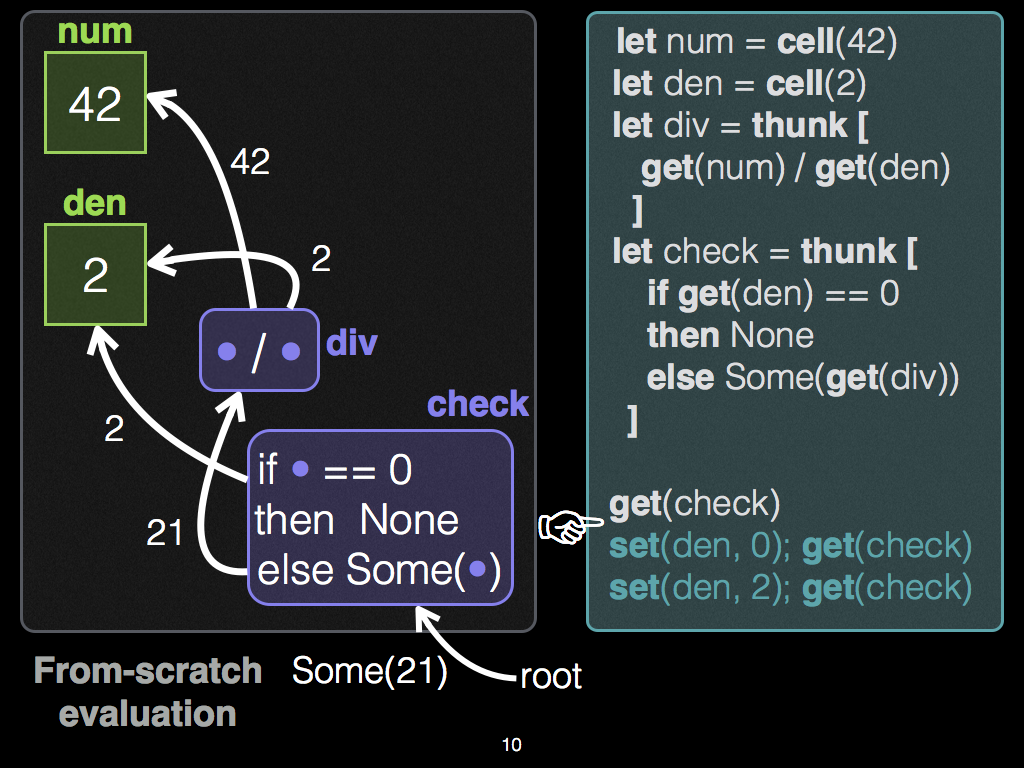Editor Steps 2 and 3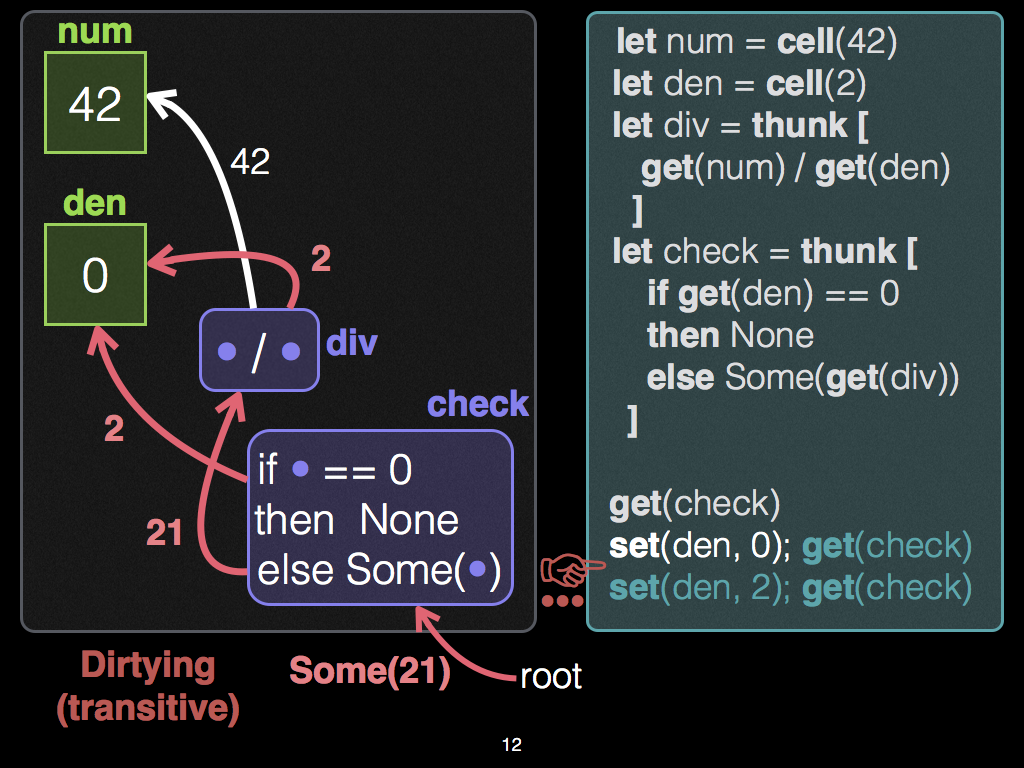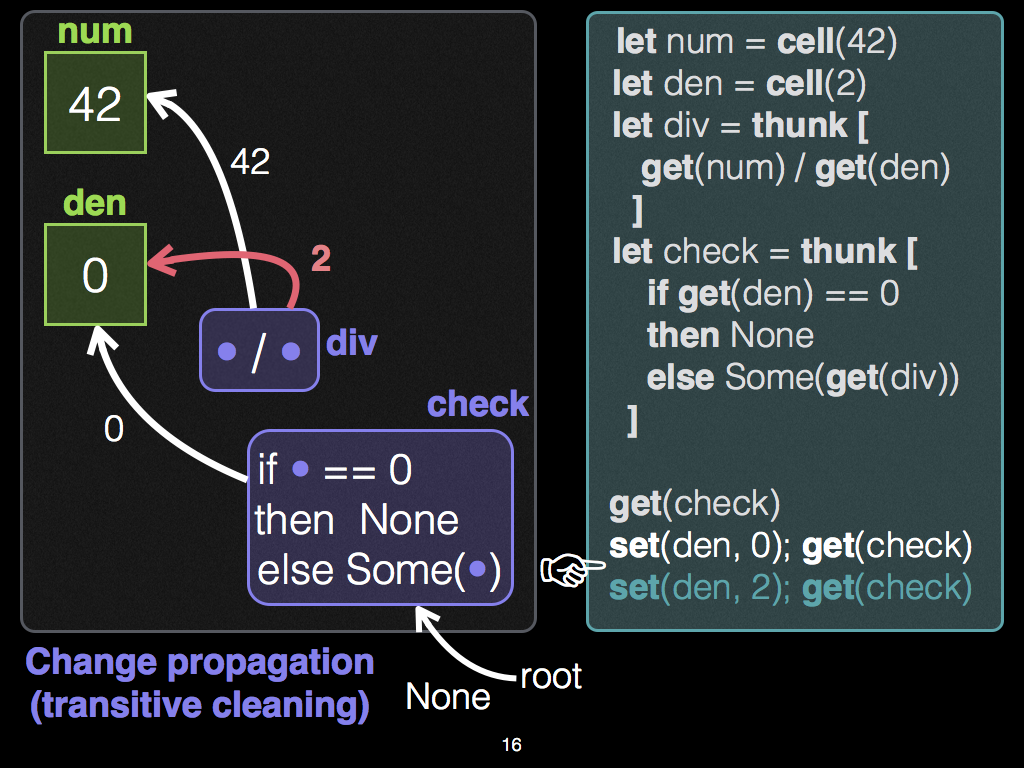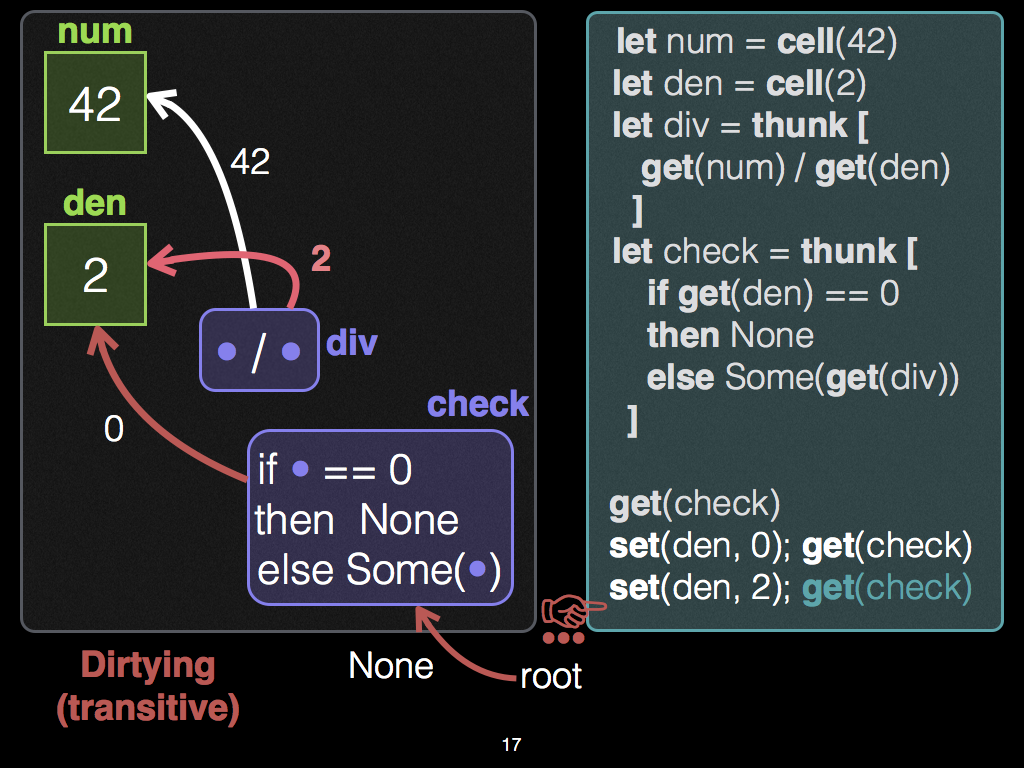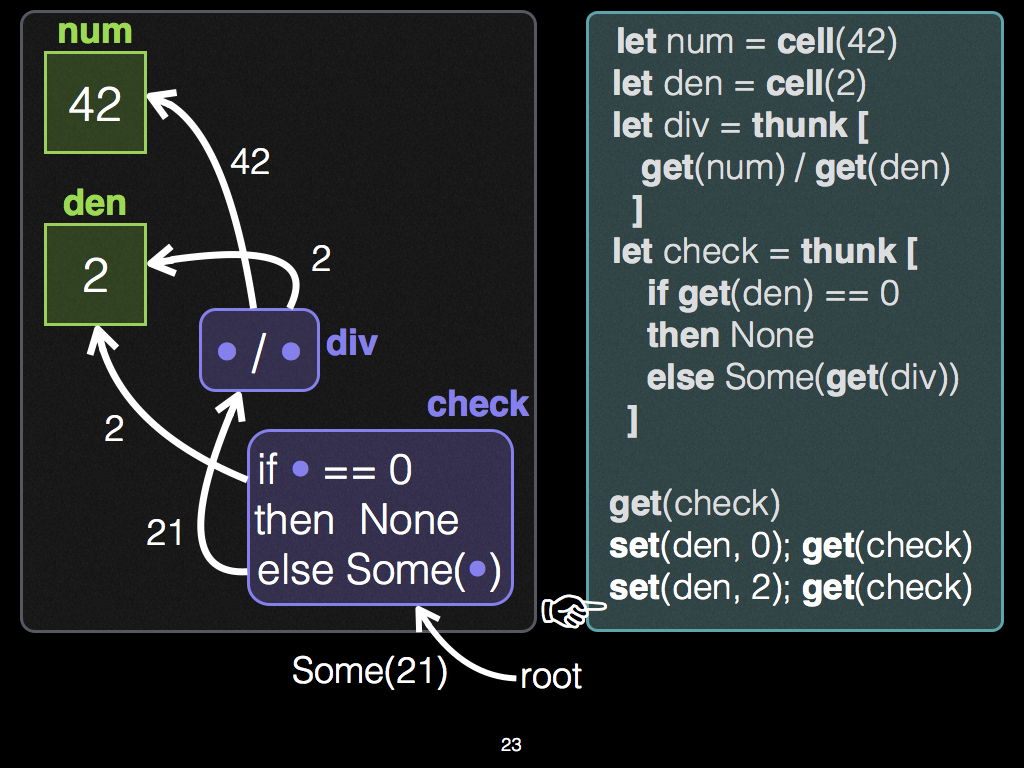Full-sized slides

In sum, whether div runs is based on demand from the Editor (of check), and the value of input den. The reuse of div illustrates the switching pattern, which is unique to Adapton's approach to incremental computation.

Switching

In the academic literature on Adapton, we refer to the three-step pattern of change propagation illustrated above as switching:

Past work on self-adjusting computation does not support the switching pattern directly: Because of its change propagation semantics, it would "forget" the division in step 2, and rerun it from-scratch in step 3.

Furthermore, some other change propagation algorithms base their re-execution schedule on "node height" (of the graph's topological ordering). These algorithms may also have undesirable behavior. In particular, they may re-execute the division div in step 2, though it is not presently in demand. For an example, see this gist.

Memoization

Memoization provides a mechanism for caching the results of subcomputations; it is a crtical feature of Adapton's approach to incremental computation.

In Adapton, each memoization point has three ingredients:

• A function expression (of type Fn)

• Zero or more arguments. Each argument type must have an implementation for the traits Eq + Clone + Hash + Debug. The traits Eq and Clone are both critical to Adapton's caching and change propagation engine. The trait Hash is required when Adapton's naming strategy is structural (e.g., where function names are based on the hashes of their arguments). The trait Debug is useful for debugging, and reflection.

• An optional name, which identifies the function call for reuse later.

• When this optional name is None, the memoization point may be treated in one of two ways: either as just an ordinary, uncached function call, or as a cached function call that is identified structurally, by its function pointer and arguments. Adapton permits structural subcomputations via the engine's structural function.

• When this is Some(name), the memoization point uses name to identify the work performed by the function call, and its result. Critically, in future incremental runs, it is possible for name to associate with different functions and/or argument values.

Each memoization point yields two results:

• A thunk articulation, of type Art<Res>, where Res is the result type of the function expression.

• A result value of type Res, which is also cached at the articulation.

Optional name version

The following form is preferred:

memo!( [ optional_name ]? fnexp ; lab1 : arg1, ..., labk : argk )

It accepts an optional name, of type Option<Name>, and an arbitrary function expression fnexp (closure or function pointer). Like the other forms, it requires that the programmer label each argument.

Example

let (t,z) : (Art<usize>, usize) =
memo!([Some(name_unit())]?
|x:usize,y:usize|{ if x > y { x } else { y }};
x:10,   y:20   );

assert_eq!(z, 20);

More examples of memo! macro

Create thunks

Thunks consist of suspended computations whose observations, allocations and results are cached in the DCG, when forced. Each thunk has type Art<Res>, where Res is the return type of the thunk's suspended computation.

Each memoization point is merely a forced thunk. We can also create thunks without demanding them.

The following form is preferred:

thunk!( [ optional_name ]? fnexp ; lab1 : arg1, ..., labk : argk )

It accepts an optional name, of type Option<Name>, and an arbitrary function expression fnexp (closure or function pointer). Like the other forms, it requires that the programmer label each argument.

Example

let t : Art<usize> =
thunk!([ Some(name_unit()) ]?
|x:usize,y:usize|{ if x > y { x } else { y }};
x:10,   y:20   );

assert_eq!(get!(t), 20);

More examples of thunk! macro

Use force_map for more precise dependencies

Suppose that we want to project only one field of type A from a pair within an Art<(A,B)>. If the field of type B changes, our observation of the A field will not be affected.

Below, we show that using force_map prunes the dirtying phase of change propagation. Doing so means that computations that would otherwise be dirty and cleaned via re-execution are never diritied in the first place. We show a simple example of projecting a pair.

To observe this fact, this test traces the engine, counts the number of dirtying steps, and ensures that this count is zero, as expected.

// Trace the behavior of change propagation; ensure dirtying works as expected
reflect::dcg_reflect_begin();

let pair  = cell!((1234, 5678));
let pair1 = pair.clone();

let t = thunk![{
// Project the first component of pair:
let fst = force_map(&pair, |_,x| x.0);
fst + 100
}];

// The output is `1234 + 100` = `1334`
assert_eq!(get!(t), 1334);

// Update the second component of the pair; the first is still 1234
set(&pair1, (1234, 8765));

// The output is still `1234 + 100` = `1334`
assert_eq!(get!(t), 1334);

// Assert that nothing was dirtied (due to using `force_map`)
let traces = reflect::dcg_reflect_end();
let counts = reflect::trace::trace_count(&traces, None);
assert_eq!(counts.dirty.0, 0);
assert_eq!(counts.dirty.1, 0);

Nominal memoization

Adapton offers nominal memoization, which uses first-class names (each of type Name) to identify cached computations and data.

fn sum(x:usize, y:usize) -> usize {
x + y
}

// create a memo entry, named `a`, that remembers that `sum(42,43) = 85`
let res1 : usize = get!(thunk!([a] sum; x:42, y:43));

Behind the scenes, the name a controls how and when the Adapton engine overwrites the cached computation of sum. As such, names permit patterns of programmatic cache eviction.

The macro memo! relies on programmer-supplied variable names in its macro expansion of these call sites, shown as x and y in the uses above. These can be chosen arbitrarily: So long as these symbols are distinct from one another, they can be any symbols, and need not actually match the formal argument names.

Example as Editor role For a simple illustration, we memoize several function calls to sum with different names and arguments. In real applications, the memoized function typically performs more work than summing two machine words. :)

// Optional: Traces what the engine does below (for diagnostics, testing, illustration)
reflect::dcg_reflect_begin();

// create a memo entry, named `a`, that remembers that `sum(42,43) = 85`
let res1 : usize = get!(thunk!([a] sum; x:42, y:43));

// same name `a`, same arguments (42, 43), Adapton reuses cached result
let res2 : usize = get!(thunk!([a] sum; x:42, y:43));

// different name `b`, same arguments (42, 43), Adapton re-computes `sum` for `b`
let res3 : usize = get!(thunk!([b] sum; x:42, y:43));

// same name `b`, different arguments, editor overwrites thunk `b` with new args
let res4 : usize = get!(thunk!([b] sum; x:55, y:66));

Below we confirm the following facts:

• The Editor:
• allocated two thunks (a and b),
• allocated one thunk without changing it (a, with the same arguments)
• allocated one thunk by changing it (b, with different arguments)
• The Archivist allocated nothing.
// Optional: Assert what happened above, in terms of analytical counts
let traces = reflect::dcg_reflect_end();
let counts = reflect::trace::trace_count(&traces, None);

// Editor allocated two thunks (`a` and `b`)
assert_eq!(counts.alloc_fresh.0, 2);

// Editor allocated one thunk without changing it (`a`, with same args)
assert_eq!(counts.alloc_nochange.0, 1);

// Editor allocated one thunk by changing it (`b`, different args)
assert_eq!(counts.alloc_change.0, 1);

// Archivist allocated nothing
assert_eq!(counts.alloc_fresh.1, 0);

Nominal Firewalls

Nominal firewalls use nominal allocation to dirty the DCG incrementally, while change propagation cleans it.

In some situations (Run 2, below), these firewalls prevent dirtying from cascading, leading to finer-grained dependency tracking, and more incremental reuse. Thanks to @nikomatsakis for suggesting the term "firewall" in this context.

First, consider this graph, as Rust code (graph picture below):

Example: nominal firewall

fn demand_graph(a: Art<i32>) -> String {
let_memo!{
d =(f)= {
let a = a.clone();
let_memo!{ b =(g)={ let x = get!(a); cell!([b] x * x) };
c =(h)={ format!("{:?}", get!(b)) };
c }};
d }
}

The use of let_memo! is convenient sugar for thunk! and force. This code induces DCGs with the following structure:

/*                                             +---- Legend ------------------+
cell a                                         | [ 2 ]   ref cell holding 2   |
[ 2 ]            "Nominal                      |  (g)    thunk named 'g'      |
^               firewall"                    | ---->   force/observe edge   |
| force            |                         | --->>   allocation edge      |
| 2               \|/                        +------------------------------+
|                  `
|   g allocs b    cell    g forces b          When cell a changes, g is dirty, h is not;
|   to hold 4      b      observes 4          in this sense, cell b _firewalls_ h from g:
(g)------------->>[ 4 ]<--------------(h)  <~~ note that h does not observe cell a, or g.
^                                     ^
| f forces g                          | f forces h,
| g returns cell b                    | returns String "4"
|                                     |
(f)------------------------------------+
^
| force f,
| returns String "4"
|
(demand_graph(a))                                                                        */

In this graph, the ref cell b acts as the "firewall".

Below, we show a particular input change for cell a where a subcomputation h is never dirtied nor cleaned by change propagation (input change 2 to -2). We show another change to the same input where this subcomputation h is eventually dirtied and cleaned by Adapton, though not immediately (input change -2 to 3).

Here's the Rust code for generating this DCG, and these changes to its input cell, named "a":

// 1. Initialize input cell "a" to hold 2, and do the computation illustrated above:
assert_eq!(demand_graph(let_cell!{a = 2; a}), "4".to_string());

// 2. Change input cell "a" to hold -2, and do the computation illustrated above:
assert_eq!(demand_graph(let_cell!{a = -2; a}), "4".to_string());

// 3. Change input cell "a" to hold 3, and do the computation illustrated above:
assert_eq!(demand_graph(let_cell!{a = 3; a}),  "9".to_string());

Run 1. In the first computation, the input cell a holds 2, and the final result is "4".

Run 2. When the input cell a changes, e.g., from 2 to -2, thunks f and g are dirtied. Thunk g is dirty because it observes the changed input. Thunk f is dirty because it demanded (observed) the output of thunk g in the extent of its own computation.

Importantly, thunk h is not immediately dirtied when cell a changes. In a sense, cell a is an indirect ("transitive") input to thunk h. This fact may suggest that when cell a is changed from 2 to -2, we should dirty thunk h immediately. However, thunk h is related to this input only by reading ref cell b.

Rather, when the editor re-demands thunk f, Adapton will necessarily perform a cleaning process (aka, "change propagation"), re-executing g, its immediate dependent, which is dirty. Since thunk g merely squares its input, and 2 and -2 both square to 4, the output of thunk g will not change in this case. Consequently, the observers of cell b, which holds this output, will not be dirtied or re-executed. In this case, thunk h is this observer. In situations like these, Adapton's dirtying + cleaning algorithms do not dirty nor clean thunk h.

In sum, under this change, after f is re-demanded, the cleaning process will first re-execute g, the immediate observer of cell a. Thunk g will again allocate cell b to hold 4, the same value as before. It also yields this same cell pointer (to cell b). Consequently, thunk f is not re-executed, and is cleaned. Meanwhile, the outgoing (dependency) edges thunk of h are never dirtied. Effectively, the work of h is reused from cache as well.

Alternatively, if we had placed the code for format!("{:?}",get!(b)) in thunk f, Adapton would have re-executed this step when a changes from 2 to -2: It would be dirtied when a changes, since it directly observes g, which directly observes cell a.

Run 3. For some other change, e.g., from 2 to 3, thunk h would eventually will be

• dirtied, when f redemands g, which will overwrite cell b with 9,
• and cleaned, when f re-demands h, which will format! a new String of "9".

let_memo! example

The use of let_memo! macro above expands as follows:

fn demand_graph__mid_macro_expansion(a: Art<i32>) -> String {
let f = thunk!([f]{
let a = a.clone();
let g = thunk!([g]{ let x = get!(a);
cell!([b] x * x) });
let b = force(&g);
let h = thunk!([h]{ let x = get!(b);
format!("{:?}", x) });
let c = force(&h);
c });
let d = force(&f);
d
};

Incremental sequences

A level tree consists of a binary tree with levels that decrease monotonically along each path to its leaves.

Here, we implement incremental level trees by including Names and Arts in the tree structure, with two additional constructors for the recursive type, Rec<X>:

use std::fmt::Debug;
use std::hash::Hash;

#[derive(Clone,PartialEq,Eq,Debug,Hash)]
enum Rec<X> {
Bin(BinCons<X>),
Leaf(LeafCons<X>),
Name(NameCons<X>),
Art(Art<Rec<X>>),
}

#[derive(Clone,PartialEq,Eq,Debug,Hash)]
struct LeafCons<X> {
elms:Vec<X>,
}

#[derive(Clone,PartialEq,Eq,Debug,Hash)]
struct BinCons<X> {
level: u32,
recl:Box<Rec<X>>,
recr:Box<Rec<X>>
}

#[derive(Clone,PartialEq,Eq,Debug,Hash)]
struct NameCons<X> {
level:u32,
name:Name,
rec:Box<Rec<X>>,
}

Example: Nominal memoization and recursion

Introduction forms:

impl<X:'static+Clone+PartialEq+Eq+Debug+Hash>
Rec<X> {

pub fn leaf(xs:Vec<X>) -> Self {
Rec::Leaf(LeafCons{elms:xs})
}
pub fn bin(lev:u32, l:Self, r:Self) -> Self {
Rec::Bin(BinCons{level:lev,recl:Box::new(l),recr:Box::new(r)})
}
pub fn name(lev:u32, n:Name, r:Self) -> Self {
Rec::Name(NameCons{level:lev,name:n, rec:Box::new(r)})
}
fn art(a:Art<Rec<X>>) -> Self {
Rec::Art(a)
}

Elimination forms: Folds use memo! to create and force thunks:

pub fn fold_monoid<B:'static+Clone+PartialEq+Eq+Debug+Hash>
(t:Rec<X>, z:X, b:B,
bin:fn(B,X,X)->X,
art:fn(Art<X>,X)->X)
-> X
{
fn m_leaf<B:Clone,X>(m:(B,fn(B,X,X)->X,X), elms:Vec<X>) -> X {
// ...
}
fn m_bin<B,X>(_n:Option<Name>, m:(B,fn(B,X,X)->X,X), _lev:u32, l:X, r:X) -> X {
m.1(m.0, l, r)
}
Self::fold_up_namebin::<(B,fn(B,X,X)->X,X),
(B,fn(B,X,X)->X,X),X> (t, (b.clone(),bin,z.clone()), m_leaf,
None, (b,bin,z), m_bin, art)
}

fn fold_up_namebin
<L:'static+Clone+PartialEq+Eq+Debug+Hash,
B:'static+Clone+PartialEq+Eq+Debug+Hash,
R:'static+Clone+PartialEq+Eq+Debug+Hash>
(t:Rec<X>,
l:L, leaf:fn(L,Vec<X>)->R,
n:Option<Name>, b:B,
namebin:fn(Option<Name>,B,u32,R,R)->R,
art:fn(Art<R>,R)->R)
-> R
{
match t {
Rec::Art(a) => Self::fold_up_namebin(get!(a), l, leaf, n, b, namebin, art),
Rec::Leaf(leafcons) => leaf(l, leafcons.elms),
Rec::Bin(bincons)   => {
let (n1,n2) = forko!(n.clone());
let r1 = memo!([n1]? Self::fold_up_namebin;
t:*bincons.recl,
l:l.clone(), leaf:leaf, n:None, b:b.clone(), namebin:namebin, art:art);
let r1 = art(r1.0,r1.1);
let r2 = memo!([n2]? Self::fold_up_namebin;
t:*bincons.recr,
l:l.clone(), leaf:leaf, n:None, b:b.clone(), namebin:namebin, art:art);
let r2 = art(r2.0,r2.1);
namebin(n, b, bincons.level, r1, r2)
}
Rec::Name(namecons) => {
Self::fold_up_namebin(
*namecons.rec,
l, leaf, Some(namecons.name), b, namebin, art
)
}
}
}

Modules

 catalog Experimental collections, including those for sequences and sets. (See also: The IODyn crate) engine Adapton's core calculus, implemented as a runtime library. We implement two versions of this interface, which we refer to as engines: The naive engine and the DCG engine, implemented based on the algorithms from the Adapton papers. macros Macros to make using the engine module's interface more ergonomic. parse_val Parses the output of Rust Debug strings into reflected values of type Val. (See engine::reflect module). reflect Reflects the DCG engine, including both the effects of the programs running in it, and the internal effects of the engine cleaning and dirtying the DCG. For the latter effects, see the trace module.

Macros

 cell Convenience wrappers for engine::cell. fork Wrappers for engine::fork_name. forko Optional name forking. get Convenience wrapper for engine::force let_cell Let-bind a nominal ref cell via cell, using the let-bound variable identifier as its name. Permits sequences of bindings. let_memo Let-bind a nominal thunk, force it, and let-bind its result. Permits sequences of bindings. let_thunk Let-bind a nominal thunk via thunk!, without forcing it. Permits sequences of bindings. memo Wrappers for creating and forcing thunks (engine::thunk and engine::force). thunk Wrappers for engine::thunk.